1. /
2. CBSE
3. /
4. Class 06
5. /
6. Mathematics
7. /
8. NCERT Solutions for Class...

# NCERT Solutions for Class 6 Maths Exercise 5.8

NCERT solutions for Maths Understanding elementary## NCERT Solutions for Class 6 Maths Understanding elementary

##### Question 1.Examine whether the following are polygons. If anyone among these is not, say why?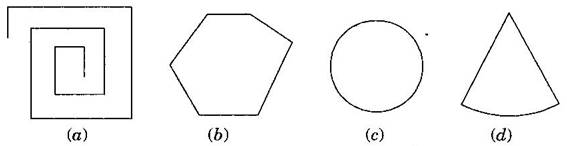(a) As it is not a closed figure, therefore, it is not a polygon.

(b) It is a polygon because it is closed by line segments.

(c) It is not a polygon because it is not made by line segments.

(d) It is not a polygon because it not made only by line segments, it has curved surface also.

###### Question 2.Name each polygon: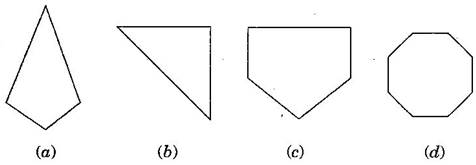(a) Quadrilateral, (b) Triangle, (c) Pentagon, (d) Octagon

NCERT Solutions for Class 6 Maths Exercise 5.8

###### Question 3.Draw a rough sketch of a regular hexagon. Connecting any three of its vertices, draw a triangle. Identify the type of the triangle you have drawn.

Answer: ABCDEF is a regular hexagon and triangle thus formed by joining AEF is an isosceles triangle.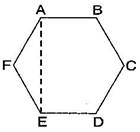###### Question 4.Draw a rough sketch of a regular octagon. (Use squared paper if you wish). Draw a rectangle by joining exactly four of the vertices of the octagon.

Answer: ABCDEFGH is a regular octagon and CDGH is a rectangle.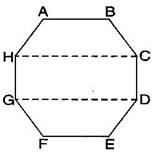NCERT Solutions for Class 6 Maths Exercise 5.8

###### Question 5.A diagonal is a line segment that joins any two vertices of the polygon and is not a side of the polygon. Draw a rough sketch of a pentagon and draw its diagonals.

Answer: ABCDE is the required pentagon and its diagonals are AD, AC, BE and BD.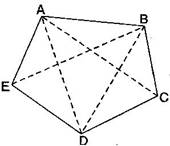## NCERT Solutions for Class 6 Maths Exercise 5.8

NCERT Solutions Class 6 Maths PDF (Download) Free from myCBSEguide app and myCBSEguide website. Ncert solution class 6 Maths includes text book solutions from Class 6 Maths Book . NCERT Solutions for CBSE Class 6 Maths have total 14 chapters. 6 Maths NCERT Solutions in PDF for free Download on our website. Ncert Maths class 6 solutions PDF and Maths ncert class 6 PDF solutions with latest modifications and as per the latest CBSE syllabus are only available in myCBSEguide.

## CBSE app for Students

To download NCERT Solutions for Class 6 Maths, Social Science Computer Science, Home Science, Hindi English, Maths Science do check myCBSEguide app or website. myCBSEguide provides sample papers with solution, test papers for chapter-wise practice, NCERT solutions, NCERT Exemplar solutions, quick revision notes for ready reference, CBSE guess papers and CBSE important question papers. Sample Paper all are made available through the best app for CBSE students and myCBSEguide website.Test Generator

Create question paper PDF and online tests with your own name & logo in minutes.myCBSEguide

Question Bank, Mock Tests, Exam Papers, NCERT Solutions, Sample Papers, Notes

### 2 thoughts on “NCERT Solutions for Class 6 Maths Exercise 5.8”

1. This app is very good but I don’t know how to install this app thanks.